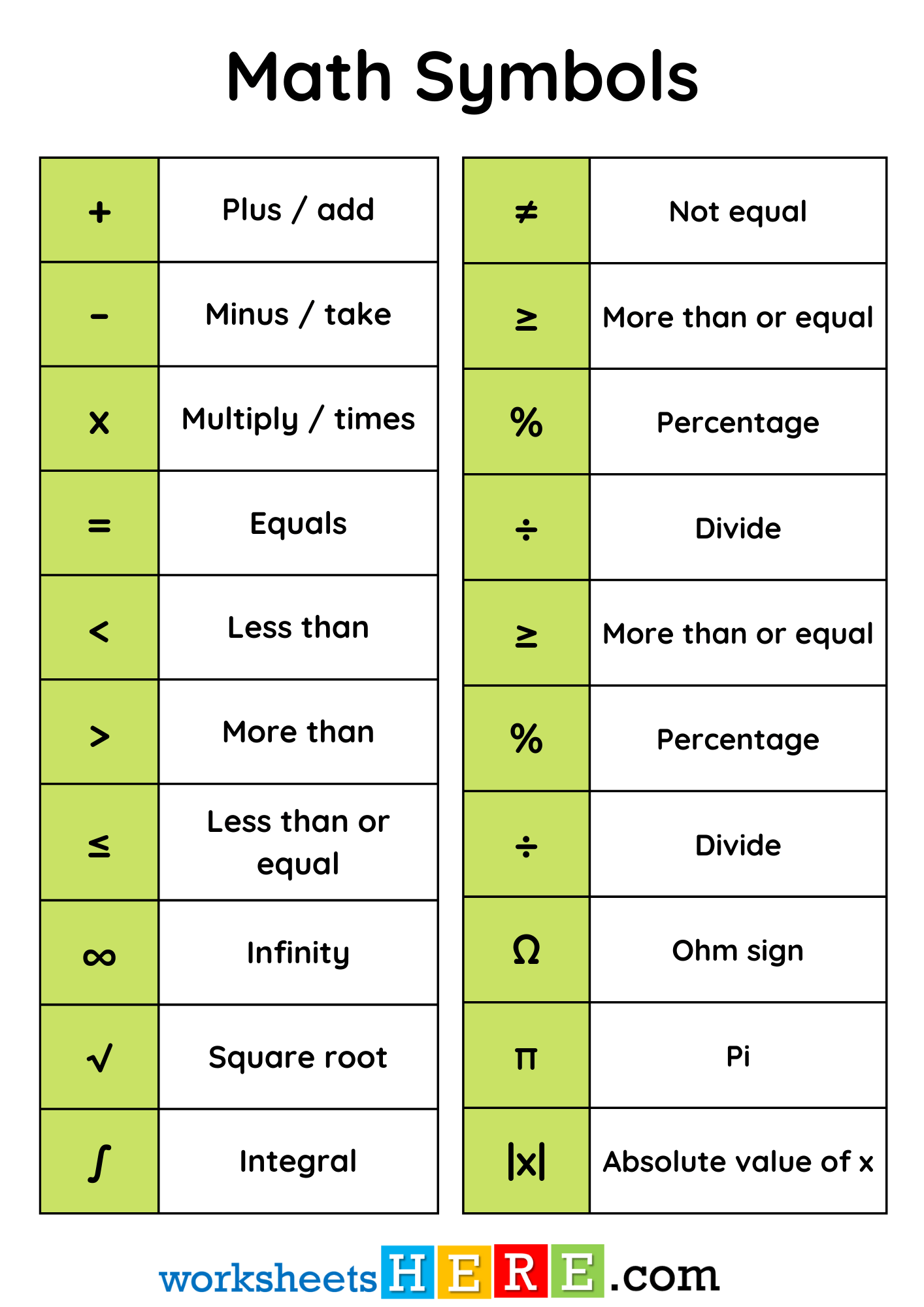# Math Symbols and Meaning PDF Worksheet For Students and Kids

Math Symbols and Meaning PDF Worksheet For Students and KidsFor Download PDF Worksheet, Click Here; Math Symbols and Meaning PDF Worksheet For Students and Kids

 + Plus / add – Minus / take x Multiply / times = Equals < Less than > More than ≤ Less than or equal ∞ Infinity √ Square root ∫ Integral
 ≠ Not equal ≥ More than or equal % Percentage ÷ Divide ≥ More than or equal % Percentage ÷ Divide Ω Ohm sign π Pi |x| Absolute value of x